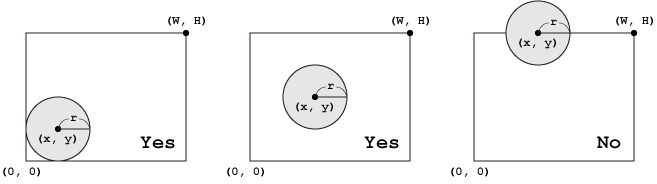# Branch on Condition - Circle in a Rectangle

Time Limit : 1 sec, Memory Limit : 131072 KB
Japanese version is here

# Circle in a Rectangle

Write a program which reads a rectangle and a circle, and determines whether the circle is arranged inside the rectangle. As shown in the following figures, the upper right coordinate $(W, H)$ of the rectangle and the central coordinate $(x, y)$ and radius $r$ of the circle are given.## Input

Five integers $W$, $H$, $x$, $y$ and $r$ separated by a single space are given in a line.

## Output

Print "Yes" if the circle is placed inside the rectangle, otherwise "No" in a line.

## Constraints

• $-100 \leq x, y \leq 100$
• $0 < W, H, r \leq 100$

## Sample Input 1

5 4 2 2 1


## Sample Output 1

Yes


## Sample Input 2

5 4 2 4 1


## Sample Output 2

No